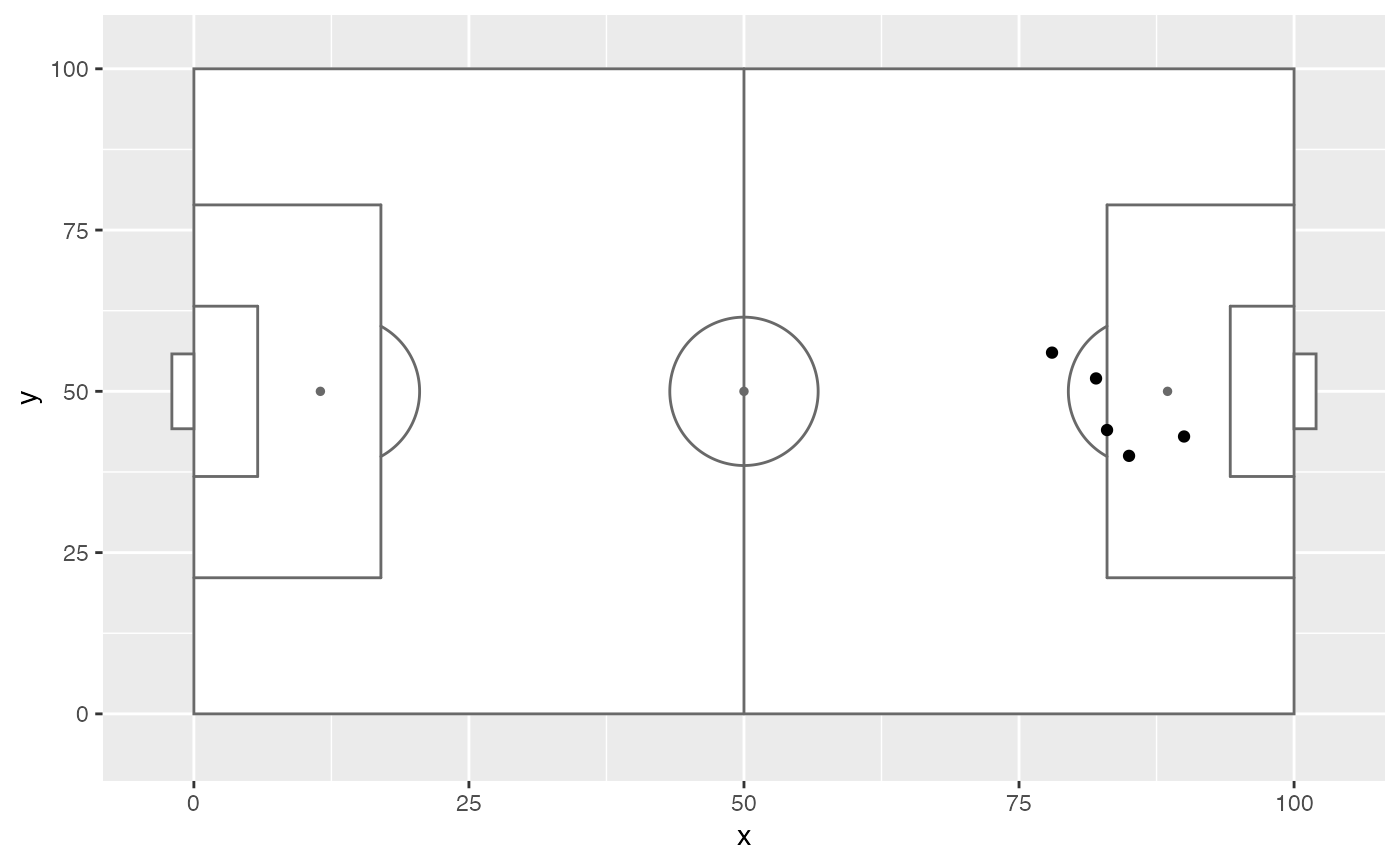Adds soccer pitch markings as a layer for use in a ggplot plot.

annotate_pitch(
colour = "dimgray",
fill = "white",
limits = TRUE,
dimensions = pitch_opta,
goals = goals_box
)

Arguments

colour

Colour of pitch outline.

fill

Colour of pitch fill.

limits

Whether to adjust the plot limits to display the whole pitch.

dimensions

A list containing the pitch dimensions to draw. See help(pitch_opta).

goals

A function for generating goal markings. Defaults to goals_box. See help(goals_box). Formulas are turned into functions with rlang::as_function.

Value

list of ggplot geoms to be added to a ggplot plot

Examples

shots_data <- data.frame(x = c(90, 85, 82, 78, 83),
y = c(43, 40, 52, 56, 44))

ggplot(shots_data, aes(x = x, y = y)) +
annotate_pitch() +